Refer to our Texas Go Math Grade 7 Answer Key Pdf to score good marks in the exams. Test yourself by practicing the problems from Texas Go Math Grade 7 Lesson 5.1 Answer Key Probability.

Finding the Likelihood of an Event

Each time you roll a number cube, a number from 1 to 6 lands face up. This is called an event.

Work with a partner to decide how many of the six possible results of rolling a number cube match the described event.

Then order the events from least likely (1) to most likely (9) by writing a number in each box to the right.

Rolling a number less than 7 ______________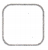Rolling an 8 ______________Rolling a number greater than 4 ______________Rolling a 5 ______________Rolling a number other than 6. ______________Rolling an even number ______________Rolling a number less than 5 ______________Rolling an odd number ______________Rolling a number divisible by 3. ______________Reflect

Question 1.
Are any of the events impossible?.
Rolling an 8 is impossible because the number cube has numbers from 1 to 6.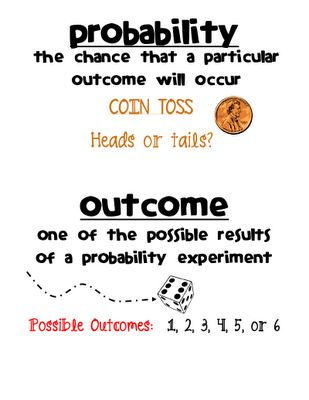The probability of event A is $$\frac{1}{3}$$. The probability of event B is $$\frac{1}{4}$$. What can you conclude about the two events?
The probability od event A is lower than $$\frac{1}{2}$$, so this event is unlikely to happen.
Because od the same reason, event B is also unlikely to happen.
But, $$\frac{1}{3}$$ is greater than $$\frac{1}{4}$$, se event A is more likely to happen than event B.

Question 3.
A hat contains pieces of paper marked with the numbers 1 through 16. Tell whether picking an even number is impossible, unlikely, as likely as not, likely, or certain. Tell whether the probability is 0, close to 0, $$\frac{1}{2}$$, close to 1, or 1.
This event is as likely as not
The probability is $$\frac{1}{2}$$.

Question 4.
Picking a purple marble from a jar with 10 green and 10 purple marbles. ____________________
Jar has 20 marbles, ten green, and ten purple.The probability of picking a purple marble is $$\frac{1}{2}$$.

Question 5.
Rolling a number greater than 4 on a standard number cube.
Samp[e space for a standard number cube is {1, 2, 3, 4, 5, 6}.The probability of rolling a number greater than 4 is $$\frac{1}{3}$$.

Reflect

Question 6.
Why do the probability of an event and the probability of its complement add up to 1 ?
The probability of an event and the probability of its complement add up to 1 because the total events are the even itself and its compliment, so its probability equal to 1 because these are all the possible events that will. occur.

A jar contains 8 marbles marked with the numbers 1 through 8. You pick a marble at random. What is the probability of not picking the marble marked with the number 5? __________
Sum of probability from some event and his complement is equal 1.
P(Event) + P(Complement) = 1
Event in this problem is picking the marble with number five.
Complement is not to pick marble with number five.
P(Picking marble with number five) + P(Complement) = 1
We have 8 marbles.Probability to pick marble with number 5 is $$\frac{1}{8}$$.
$$\frac{1}{8}$$ + P(Complement) = 1
Write one as $$\frac{8}{8}$$.
Subtract $$\frac{1}{8}$$ from both sides in equality.
$$\frac{1}{8}$$ + P(Complement) – $$\frac{1}{8}$$ = $$\frac{8}{8}$$ – $$\frac{1}{8}$$
P(Not to pick marble with number five) = $$\frac{7}{8}$$

Question 8.
You roll a standard number cube. Use the probability of rolling an even number to find the probability of rolling an odd number. __________
The sum of the probabiLities of an event and its complement equals 1.
First, find the probability of rolling an even number
Sample space for a standard number cube is {1, 2. 3, 4, 5, 6} and number of ways to roll an even number is 3.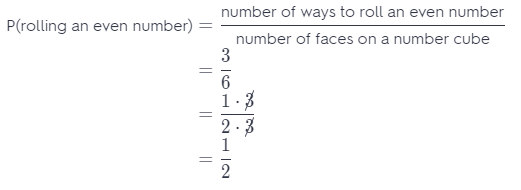The probability of rolling an even number is $$\frac{1}{2}$$.
The probability of not rolling an even number is the same as the probability of rolling an odd number.
Hence,
P(even number) + P(odd number) = 1 Substitute $$\frac{1}{2}$$ for P(even number)
$$\frac{1}{2}$$ + P(odd number) = 1 Subtract $$\frac{1}{2}$$ from both sides
P(odd number) = 1 – $$\frac{1}{2}$$
P(odd number) = $$\frac{1}{2}$$
The probability of rolling an odd number is $$\frac{1}{2}$$.

Question 1.
In a hat, you have index cards with the numbers 1 through 10 written on them. Order the events from least likely to happen (1) to most likely to happen (8) when you pick one card at random. In the boxes, write a number from 1 to 8 to order the eight different events. (Explore Activity)
You pick a number greater than 0.You pick an even number.You pick a number that is at least 2.You pick a number that ¡s at most 0.You pick a number divisible by 3.You pick a number divisible by 5.You pick a prime number.You pick a number less than the greatest prime number.Write events from Least likeLy to happen to the most likely to happen.

The least likely to happen event is that you pick a number that is the most 0, because all of the numbers from 1 to 10 are greater than 0.

Next is event that you pick a number divisible by 5, because there is only 2 numbers (10, 5) from 1 to 10 that is divisible by 5

Next is event that you pick a number divisible by 3, because there is 3 numbers (3, 6, 9) from 1 to 10 that is divisible by 3
Next is event that you pick a prime number, because there is 4 prime numbers (2, 3, 5, 7) from 1 to 10.

Next is event that you pick a even number, because there is 5 prime numbers (2, 4, 6. 8. 10) from 1 to 10.

Next is event that you pick a number less than the greatest prime number, because the greatest prime number from 1 to 10 is 7 and there is 6 numbers (1, 2, 3, 4, 5, 6) from 1 to 10 Less than 7.

Next is event that you pick a number that is the least 2, because except number 1 all of the numbers from 1 to 10, are at least 2.

The most likely to happen event is that you pick a number greater than 0, because all the numbers from 1 to 10, are greater than 0.
8, 5, 7, 1, 3, 2, 4, 6

Determine whether each event is impossible, unlikely, as likely as not, likely, or certain. Then, tell whether the probability is 0, close to 0, $$\frac{1}{2}$$, close to 1, or 1. (Example 1)

randomly picking a green card from a standard deck of playing cards.
Event is impossible, because there is no green cards in standard deck of playing cards.
The probability is 0.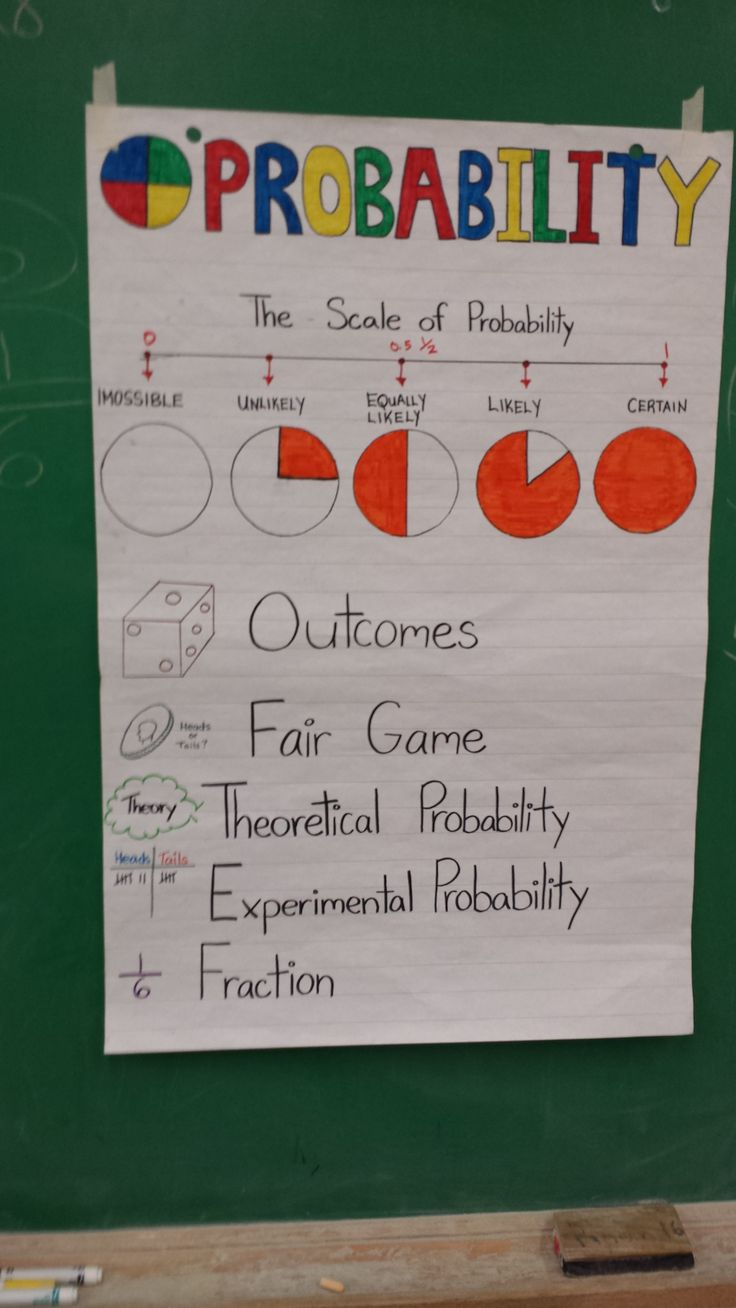Question 3.
randomly picking a red card from a standard deck of playing cards
There is 26 red cards in standard deck of playing cards.
Find the probability of picking red card from the standard deck of playing cards.= $$\frac{26}{52}$$
= $$\frac{1 \cdot 26}{2 \cdot 26}$$
= $$\frac{1}{2}$$
The probabiLity of picking red card from the standard deck of playing cards is $$\frac{1}{2}$$.
Event is as likely as not.

Question 4.
picking a number less than 15 from a jar with papers labeled from 1 to 12
Event is certain because all the numbers from 1 to 12 are less than 15.
Hence, the probability is 1.

Question 5.
picking a number that is divisible by 5 from a jar with papers labeled from 1 to 12
There is 2 numbers from 1 to 12 that are divisible by 5, and that numbers are 5 and lo.
Find the probability of picking a number that is divisible by 5.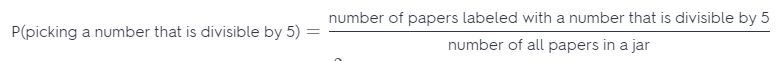= $$\frac{2}{12}$$
= $$\frac{1 \cdot 2}{6 \cdot 2}$$
= $$\frac{1}{6}$$
The probability of picking a number that is divisible by 5 is $$\frac{1}{6}$$.
This event is unlikely to occur.

Find each probability. Write your answer in the simplest form. (Example 2)

Algebra 1 with Probability Answer Key Go Math Grade 7 Question 6.
Spinning a spinner that has 5 equal sections marked 1 through 5 and landing on an even number. Use a tree diagram to find the sample space.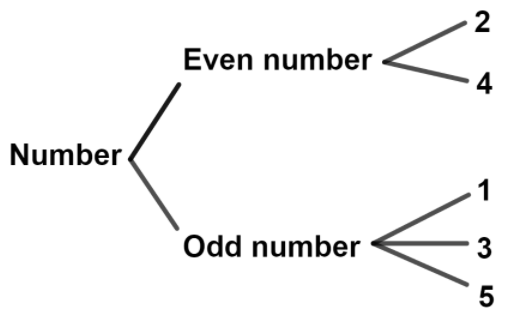P(land on even number) = $$\frac{2}{5}$$

Question 7.
Picking a diamond from a standard deck of playing cards which has 13 cards in each of four suits: spades, hearts, diamonds and clubs.
A standard deck of playing cards has 13 cards in diamonds.
Find the probability of picking a diamond.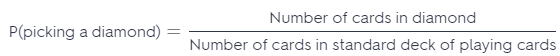= $$\frac{13}{52}$$
= $$\frac{1 \cdot 13}{4 \cdot 13}$$
= $$\frac{1}{4}$$
The probability of picking a diamond from a standard deck of playing cards is $$\frac{1}{4}$$.

Use the complement to find each probability. (Example 3)

Question 8.
What is the probability of not rolling a 5 on a standard number cube?
The sum of the probabilities of an event and ¡ts complement equals 1.
First, find the probability of rolling a number 5 on a standard number cube.
Sample space for a standard number cube is {1, 2, 3, 4, 5, 6}.= $$\frac{1}{6}$$
The probability of rolling a number 5 is $$\frac{1}{6}$$.
P(number 5) + P(not number 5) = 1 Substitute $$\frac{1}{6}$$ for P(number 5)
$$\frac{1}{6}$$ + P(not number 5) = 1 Subtract $$\frac{1}{6}$$ from both sides.
P(not number 5) = 1 – $$\frac{1}{6}$$
P(not number 5) = $$\frac{5}{6}$$
The probability of not rolling number 5 is $$\frac{5}{6}$$.
This event is likely to occur.

Question 9.
A spinner has 3 equal sections that are red, white, and blue. What is the probability of not landing on blue?
The sum of the probabilities of an event and its compLement equals 1.
First, find the probability of landing on red or white section. The sum of the red and white section is 2.
P(red or white section) =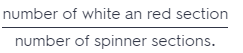= $$\frac{2}{3}$$
The probability of landing on red or white section is $$\frac{2}{3}$$.
The probability of not landing on red or white section is the same as probability or landing on blue section
P(not red or white section) = P(blue section)

P(red or white) + P(not red or white) = 1 Substitute P(blue) for P(not red or white)
P(red or white section) + P(blue section) = 1 Substitute $$\frac{2}{3}$$ for P(red or white section).
$$\frac{2}{3}$$ + P(blue section) = 1 Subtract $$\frac{2}{3}$$ from both sides.
P(blue section) = 1 – $$\frac{2}{3}$$
P(blue section) = $$\frac{1}{3}$$
The probability of landing on blue section is $$\frac{1}{3}$$.
This event is unlikely to occur.

Question 10.
A spinner has 5 equal sections marked 1 through 5. What is the probability of not landing on 4?
The sum of the probabilities of an event and its complement equals 1.
P(event) + P(complement) = 1
First, find the probabiLity of Landing on section 4.
The spinner has 5 sections with numbers 1, 2, 3, 4 and 5, hence
P(section 4) = $$\frac{1}{5}$$
P(section 4) + P(not section 4) = 1 Substitute $$\frac{1}{5}$$ for P(section 4).
$$\frac{1}{5}$$ + P(not section 4) = 1 Subtract $$\frac{1}{5}$$ from both sides.
P(not section 4) = 1 – $$\frac{1}{5}$$
P(not section 4) = $$\frac{4}{5}$$
The probability of not landing on section 4 is $$\frac{4}{5}$$.
This event is likely to occur.

There are 4 queens in a standard deck of 52 cards. You pick one card at random. What is the probability of not picking a queen?
The stint of the probabilities of an event and its complement equals 1.
P(event) + P(complement) = 1
A standard deck of playing cards has 1 queen.
Find the Probability of picking a queen.
P(queen) == $$\frac{4}{52}$$
= $$\frac{1 \cdot 4}{13 \cdot 4}$$
= $$\frac{1}{13}$$
The probability of picking a queen from a standard deck of playing cards is $$\frac{1}{13}$$
P(queen) + P(not queen) = 1 Substitute $$\frac{1}{13}$$ for P(queen).
$$\frac{1}{13}$$ + P(not queen) = 1 Subtract $$\frac{1}{13}$$ from both sides.
P(not queen) = 1 – $$\frac{1}{13}$$
P(not queen) = $$\frac{12}{13}$$
The probability of not picking a queen from a standard deck of playing cards is $$\frac{12}{13}$$.
This event is likely to occur.

Essential Question Check-In

Question 12.
Describe an event that has a probability of 0% and an event that has a probability of 100%.
Example for 0% probability: What is probability to extract cherry in a basket in which there are only strawberries.
Example for 100 % probability: Numbers on the cube are: 2, 4, 2, 4, 6, 6. What is probability to roll a an even number?

Question 13.
There are 4 aces and 4 kings in a standard deck of 52 cards. You pick one card at random. What is the probability of selecting an ace or a king? Explain your reasoning.
Find the probability of seLecting a king from a standard deck of playing cards.
P(king) =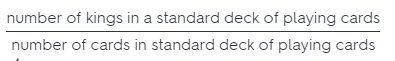= $$\frac{4}{52}$$
= $$\frac{1 \cdot 4}{13 \cdot 4}$$
= $$\frac{1}{13}$$
The probability of selecting a king from a standard deck is $$\frac{1}{13}$$
Find the probability of selecting an ace from a standard deck of playing cards.
P(ace) == $$\frac{4}{52}$$
= $$\frac{1 \cdot 4}{13 \cdot 4}$$
= $$\frac{1}{13}$$
The probability of selecting an ace from a standard deck of playing cards.$$\frac{1}{13}$$.

The probability of selecting a king or an ace is the sum of the probability of selecting a king, and the probability of selecting an ace from a standard deck
P(king or ace) = P(king) + P(ace) Substitute $$\frac{1}{13}$$ for P(king) and $$\frac{1}{13}$$ for P(ace)
P(king or ace) = $$\frac{1}{13}$$ + $$\frac{1}{13}$$
P(king or ace) = $$\frac{2}{13}$$
The probability of selecting a king or an ace is $$\frac{2}{13}$$.

There are 12 pieces of fruit in a bowl. Seven of the pieces are apples and two are peaches. What is the probability that a randomly selected piece of fruit will not be an apple or a peach? Justify your answer.
There is 7 pieces of apple and 2 pieces of peach in a bowl
Find the probability of selecting a piece of apple from a bowl
P(apple) =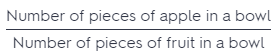= $$\frac{7}{12}$$
The probability of selecting a piece of apple from a bowl is $$\frac{7}{12}$$
Find the probability of selecting a piece of peach from a bowl.
P(peach) == $$\frac{2}{12}$$
The probability of selecting a piece of peach from a bowl is $$\frac{2}{12}$$.

The probability of selecting a piece of apple or peach from the bowl is the sum of the probability of selecting an apple, and the probability of selecting a peach from a bowl.
P(apple or peach) = P(apple) + P(peach) Substitute $$\frac{7}{12}$$ for P(apple) and $$\frac{2}{12}$$ for P(peach)
P(apple or peach) = $$\frac{7}{12}$$ + $$\frac{2}{12}$$
P(apple or peach) = $$\frac{9}{12}$$
P(apple or peach) = $$\frac{3 \cdot 3}{4 \cdot 3}$$
P(apple or peach) = $$\frac{3}{4}$$
The probability of selecting apple or peach from a bowl is $$\frac{3}{4}$$.

The sum of the probability of an event and its complement equals 1.
P(event) + P(complement) = 1
P(apple or peach) + P(not appLe or peach) = 1 Substitute $$\frac{3}{4}$$ for P(apple or peach).
$$\frac{3}{4}$$ + P(not apple or peach) = 1 Subtract $$\frac{3}{4}$$ from both sides
P(not apple or peach) = 1 – $$\frac{3}{4}$$
P(not apple or peach) = $$\frac{1}{4}$$
The probabiLity of not selecting piece of apple or peach is $$\frac{1}{4}$$.

Question 15.
Critique Reasoning For breakfast, Clarissa can choose from oatmeal, cereal, French toast, or scrambled eggs. She thinks that if she selects a breakfast at random, it is likely that it will be oatmeal. Is she correct? Explain your reasoning. Use a tree diagram to determine your sample space.
They are equally Likely to choose every dish.Question 16.
Draw Conclusions A researcher’s garden contains 90 sweet pea plants, which have either white or purple flowers. About 70 of the plants have purple flowers, and about 20 have white flowers. Would you expect that one plant randomly selected from the garden will have purple or white flowers? Explain.Garden contains 90 plants of which about 70 are with purple flowers, and about 20 with white flowers.
Find the probability of selecting the plant with purple flowers.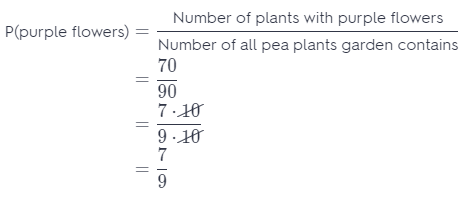The probability of selecting the plant with purple flowers is $$\frac{7}{9}$$.
Find the probability of selecting the plant with white flowers.The probability of selecting the plant with white flowers is $$\frac{2}{9}$$.

The probability of selecting the plant with purple flowers is greater than the probability of selecting the plant with white flowers, hence, when randomly select plant we expect that the plant will have purple flowers.

Question 17.
The power goes out as Sandra is trying to get dressed. If she has 4 white T-shirts and 10 colored T-shirts in her drawer, is it likely that she will pick a colored T-shirt in the dark? What is the probability she will pick a colored T-shirt? Explain your answers.
Sandra has 10 color T-shirts, and 4 white T-shirts.
Total number of T-shirts is 4 + 10 = 14
Find the probability of picking a white T-shirts.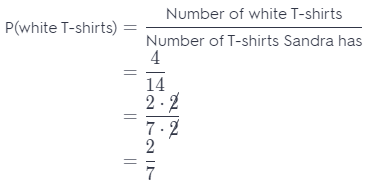The probability of picking a white T-shirts is $$\frac{2}{7}$$.
Find the probability of picking a color T-shirts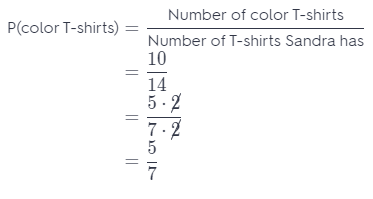The probability of picking a color T-shirts is $$\frac{5}{7}$$.

The probability of picking a color T-shirts is greater than the probability of picking a white T-shirts, hence, it is likely that she will pick a color T-shirt in the dark.

Question 18.
James counts the hair colors of the 22 people in his class, including himself. He finds that there are 4 people with brown hair, 8 people with brown hair, and 10 people with black hair. What is the probability that a randomly chosen student in the class does not have red hair? Explain.
None of the students have red hair, so the probability of choosing a student with red hair is 0. The complement of this event is the probability of choosing a student who does not have red hair.
The sum of the probability of an event and its complement equals 1.
P(red hair) + P(not red hair) = 1 Substitute 0 for P(red hair)
0 + P(not red hair) = 1
P(not red hair) = 1
The probability of choosing a student who does not have red hair is 1.
Hence, this event is certain.Question 19.
Persevere in Problem Solving A bag contains 8 blue coins and 6 red coins. A coin is removed at random and replaced by three of the other color.
a. What is the probability that the removed coin is blue?
Total number of coins in a bag is 8 + 6 = 14
Find the probability of removing blue coin from a bag.The probability of removing blue coin from a bag is $$\frac{4}{7}$$.

b. If the coin removed is blue, what is the probability of drawing a red coin after three red coins are put in the bag to replace the blue one?
If a blue coin is removed, the number of blue coins is now 8 – 1 = 7, and the total number of coins in a bag is 7 + 6 = 13.

Instead of that one blue coin that ¡s removed, we put three red coins.

Now, the total number of red coins in a bag is 6 + 3 = 9, and total number of coins in a bag is 16, 7 of blue ones, and 9 red ones coins.The probability of drawing a red coin from the bag after three red coins are put in the bag to replace the blue one is $$\frac{9}{16}$$.

c. If the coin removed is red, what is the probability of drawing a red coin after three blue coins are put in the bag to replace the red one?
c) If a red coin is removed, the number of red coins is now 6 – 1 = 5, and the total number of coins in a bag is 8 + 5 = 13.
Instead of that one red coin that is removed, we put three blue coins.
Now, the total number of bLue coins in a bag is 8 + 3 = 11, and total number of coins in a bag is 16, 11 of blue ones, and 5 red ones coins.The probability of drawing a red coin from the bag after three bLue coins are put in the bag to replace the red one $$\frac{5}{16}$$.

H.O.T. Focus on Higher Order Thinking

Question 20.
Draw Conclusions Give an example of an event in which all of the outcomes are not equally likely. Explain.
In the basket are 4 red, 2 white and 7 green balls. Find the probability for each color of a ball to be selected.Question 21.
Critique Reasoning A box contains 150 black pens and 50 red pens. Jose said the sum of the probability that a randomly selected pen will not be black and the probability that the pen will not be red is 1. Explain whether you agree.
Total number of pens in a box is 150 + 50 200.
First, find the probability of selecting black pen, and the probabality of selecting red pen.
Second, find the compliments of these two events, and check whether if their sum is 1 or not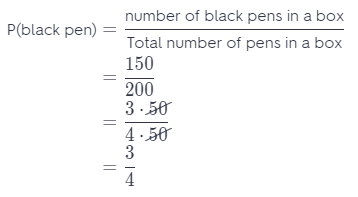The probability of selecting black pen is $$\frac{3}{4}$$.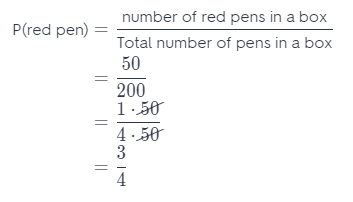The probability of selecting red pen is $$\frac{1}{4}$$.

The sum of the probabilities of an event and its complement equals 1.
P(event) + P(complement) = 1
P(black pen) + P(not black pen) = 1 Substitute $$\frac{3}{4}$$ for P(black pen).
$$\frac{3}{4}$$ + P(not black pen) = 1 Subtract $$\frac{3}{4}$$ from both sides.
P(not black pen) = 1 – $$\frac{3}{4}$$
P(not black pen) = $$\frac{1}{4}$$
The probability of not selecting black pen is $$\frac{1}{4}$$.

P(red pen) + P(not red pen) = 1 Substitute $$\frac{1}{4}$$ for P(red pen).
$$\frac{1}{4}$$ + P(not red pen) = 1 Subtract $$\frac{1}{4}$$ from both sides.
P(not red pen) = 1 – $$\frac{1}{4}$$
P(not red pen) = $$\frac{3}{4}$$
The probability of not selecting red pen is $$\frac{3}{4}$$.

P(not black) + P(not red) $$\stackrel{?}{=}$$ 1 Substitute $$\frac{1}{4}$$ for P(not black) and $$\frac{3}{4}$$ for P(not $$\frac{1}{4}+\frac{3}{4} \stackrel{?}{=} 1$$
1 $$\stackrel{?}{=}$$ 1
True
The sum of the probability that a randomly selected pen will not be black color and the probability that the pen will not be red is 1.

Question 22.
Communicate Mathematical Ideas A spinner has 7 identical sections. Two sections are blue, 1 is red, and 4 of the sections are green. Suppose the probability of an event happening is $$\frac{2}{7}$$. What does each number in the ratio represent? What outcome matches this probability?